# MySQL COUNT()

The `COUNT()` aggregate function returns the number of rows in a result set of a `SELECT` statement.

The result provided by `COUNT()` is a `BIGINT` value.

If there are no matching rows, the returned value is `0`.

Example:

Result: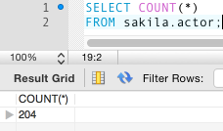This tells us that there are 204 records in the `actor` table of the `sakila` database.

If we add a `WHERE` clause, we can narrow down the result set. Like this:

Result: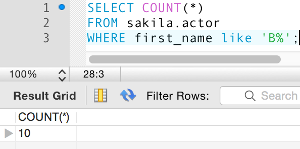The above example tells us that there are 10 records where the value of the `first_name` field begins with the letter "B".

You can see that if we remove the `COUNT(*)` function, we end up with a list of all records returned. By counting the number of rows, we see that 10 records were returned.

Result:## Variations

The `COUNT()` function can be used in different ways. You may get different results depending on how you use it, so it pays to understand how it works.

### `COUNT(expression)`

Returns the number of rows that contain non-`NULL` values.

The following example retrieves all records where the `last_name` field does not contain a `NULL` value:

### `COUNT(*)`

Returns the number of rows in a result set whether or not they contain `NULL` values.

The following example contains all records, even if some fields contain a `NULL` value. So if some actors don't have a last name recorded in the table, this statement will return a higher number than the previous example.

### `COUNT(DISTINCT expression)`

The `DISTINCT` keyword removes duplicate records. Therefore, this returns the number of unique rows that do not contain `NULL` values.

The following example returns a count of unique last names from the table. If a last name is shared by two or more actors, the result will be a lower number than the above examples.

## `COUNT()` with `GROUP BY`

You can use `COUNT()` with the `GROUP BY` clause to provide a count of records within each group.

Example:

Result: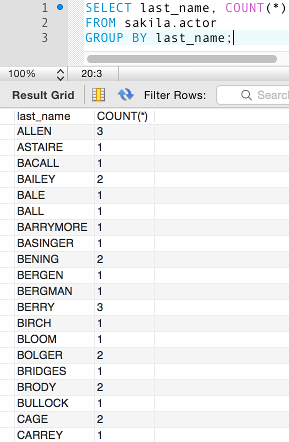The above example groups all last names and provides the count of each one. For example, we can see that three actors have `ALLEN` as their last name, only one has `ASTAIRE`, etc

### The `HAVING` Clause

You can add the `HAVING` clause to the `GROUP BY` clause to filter the results further.

Here we add a `HAVING` clause with another `COUNT()` function to return only those records that have a count greater than 3:

Result: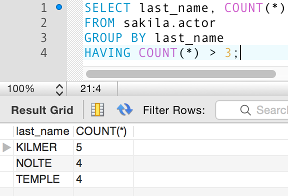## Flow Control

You can combine `COUNT()` with flow control functions for greater functionality. For example, you could use the `IF()` function as part of the expression to be used in the `COUNT()` function. Doing this can be quite helpful for providing a quick breakdown of the data within a database.

Consider this data in the `film` table:You can see that there's a field called `length`, and each film has its length recorded in that field.

So we could use that field to do the following:

Result: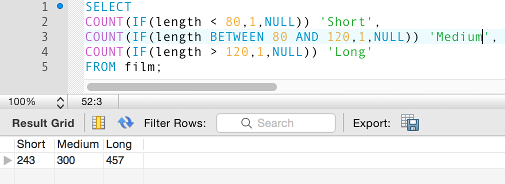The above statement separates the results into three groups; `Short`, `Medium`, and `Long`, depending on the length of the film. We use the `IF()` function to compare the length of the film to a given expression, and depending on the result, it returns a `1` or a `NULL`. If it returns a `1`, it will be counted under the column heading that we name (`Short`, `Medium`, or `Long`).